Name:    Quiz 9.3-9.4

Multiple Choice
Identify the choice that best completes the statement or answers the question.

1.

The partial tree diagram shows some of the outcomes of flipping a coin four times. How many possible outcomes are there in all?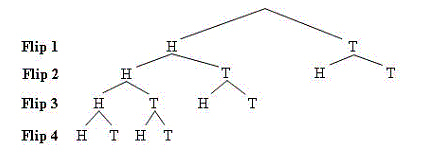a. 16 c. 12 b. 8 d. 4

2.

You write the numbers 1 through 9 on separate pieces of paper and put them in a hat. Then you draw the numbers one at a time without replacing them. What is the probability that you draw the numbers 1 through 9 in order?
 a.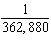c.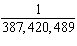b.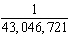d.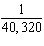3.

You have 8 pairs of socks in the dryer. Only one pair is black. You randomly choose two of the socks. What is the probability that you got both of the black socks?
 a.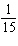c.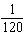b.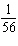d.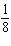Numeric Response

1.

There are 98 lettered tiles in a board game. You randomly draw the tiles shown. How many of the 98 lettered tiles would you expect to be vowels?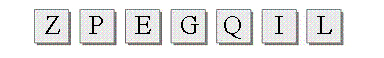2.

A baseball team has won 50% of its games so far. There are 20 regular season games left. Predict how many of these games the team will win.

3.

In a city, there are 10 motorcycles for every 50 people. Predict the number of motorcycles in a group of 290 people.

You have three sticks. Each stick has one red side and one blue side. You throw the sticks 10 times and record the results. Use the table to find the experimental probability of the event.
 Outcome Frequency 3 red 2 3 blue 0 2 blue, 1 red 5 2 red, 1 blue 3

1.

Tossing 3 blue

2.

Not tossing all red

Use the bar graph to find the experimental probability of the event.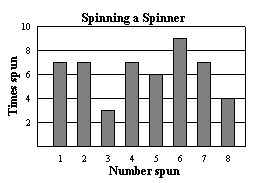3.

Not spinning a 1

Tell whether the events are independent or dependent. Explain.

4.

You flip a coin twice.
First flip: Tails

You randomly choose one of the lettered tiles. Without replacing the first tile, you choose a second tile. Find the probability of choosing the first tile, then the second tile.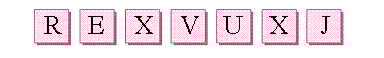5.

X and U

6.

Not X and X

7.

You are playing a shell game. A friend shuffles three shells. One of them contains a pea. You guess which shell contains the pea. If you are wrong, you guess which of the two remaining shells contains the pea. Is the probability that you find the pea on your first guess greater than the probability that you do not find the pea in two guesses? Explain.